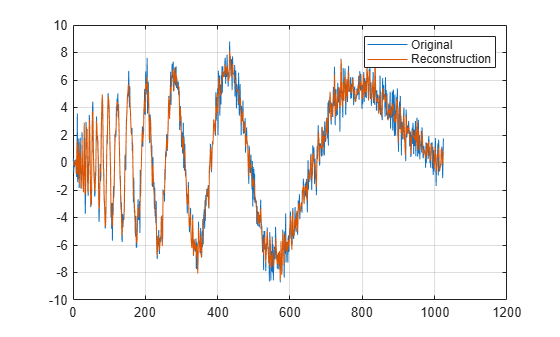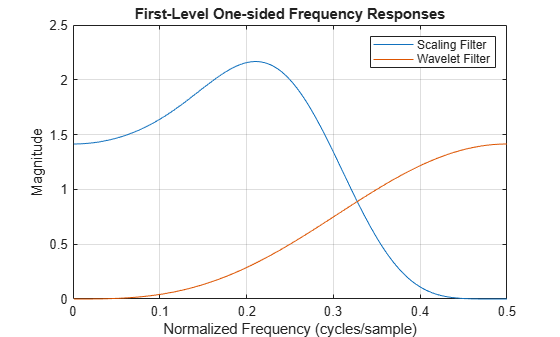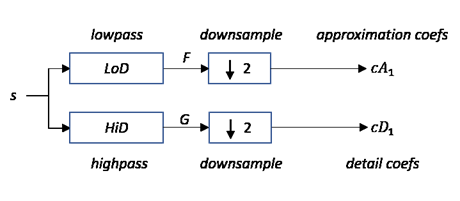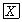# dwt

Single-level 1-D discrete wavelet transform

## Syntax

``[cA,cD] = dwt(x,wname)``
``[cA,cD] = dwt(x,LoD,HiD)``
``[cA,cD] = dwt(___,'mode',extmode)``

## Description

example

````[cA,cD] = dwt(x,wname)` returns the single-level discrete wavelet transform (DWT) of the vector `x` using the wavelet specified by `wname`. The wavelet must be recognized by `wavemngr`. `dwt` returns the approximation coefficients vector `cA` and detail coefficients vector `cD` of the DWT. NoteIf your application requires a multilevel wavelet decomposition, consider using `wavedec`. ```

example

````[cA,cD] = dwt(x,LoD,HiD)` returns the single-level DWT using the specified lowpass and highpass wavelet decomposition filters `LoD` and `HiD`, respectively.```
````[cA,cD] = dwt(___,'mode',extmode)` returns the single-level DWT with the specified extension mode `extmode`. For more information, see `dwtmode`. This argument can be added to any of the previous input syntaxes. NoteFor `gpuArray` inputs, the supported modes are `'symh'` (`'sym'`) and `'per'`. All `'mode'` options except `'per'` are converted to `'symh'`. See the example Single-Level Discrete Wavelet Transform on a GPU. ```

## Examples

collapse all

Obtain the single-level DWT of the noisy Doppler signal using a wavelet name.

```load noisdopp; [cA,cD] = dwt(noisdopp,'sym4');```

Reconstruct a smoothed version of the signal using the approximation coefficients. Plot and compare with the original signal.

```xrec = idwt(cA,zeros(size(cA)),'sym4'); plot(noisdopp) hold on grid on plot(xrec) legend('Original','Reconstruction')```Obtain the single-level DWT of a noisy Doppler signal using the wavelet (highpass) and scaling (lowpass) filters.

```load noisdopp; [LoD,HiD] = wfilters('bior3.5','d'); [cA,cD] = dwt(noisdopp,LoD,HiD);```

Create a DWT filter bank that can be applied to the noisy Doppler signal using the same wavelet. Obtain the highpass and lowpass filters from the filter bank.

```len = length(noisdopp); fb = dwtfilterbank('SignalLength',len,'Wavelet','bior3.5'); [lo,hi] = filters(fb);```

For the `bior3.5` wavelet, `lo` and `hi` are 12-by-2 matrices. `lo` are the lowpass filters, and `hi` are the highpass filters. The first columns of `lo` and `hi` are used for analysis and the second columns are used for synthesis. Compare the first column of `lo` and `hi` with `LoD` and `HiD` respectively. Confirm they are equal.

`disp('Lowpass Analysis Filters')`
```Lowpass Analysis Filters ```
`[lo(:,1) LoD']`
```ans = 12×2 -0.0138 -0.0138 0.0414 0.0414 0.0525 0.0525 -0.2679 -0.2679 -0.0718 -0.0718 0.9667 0.9667 0.9667 0.9667 -0.0718 -0.0718 -0.2679 -0.2679 0.0525 0.0525 ⋮ ```
`disp('Highpass Analysis Filters')`
```Highpass Analysis Filters ```
`[hi(:,1) HiD']`
```ans = 12×2 0 0 0 0 0 0 0 0 -0.1768 -0.1768 0.5303 0.5303 -0.5303 -0.5303 0.1768 0.1768 0 0 0 0 ⋮ ```

Plot the one-sided magnitude frequency responses of the first-level wavelet and scaling filters.

```[psidft,f,phidft] = freqz(fb); level = 1; plot(f(len/2+1:end),abs(phidft(level,len/2+1:end))) hold on plot(f(len/2+1:end),abs(psidft(level,len/2+1:end))) grid on legend('Scaling Filter','Wavelet Filter') title('First-Level One-sided Frequency Responses') xlabel('Normalized Frequency (cycles/sample)') ylabel('Magnitude')```Refer to GPU Computing Requirements (Parallel Computing Toolbox) to see what GPUs are supported.

Load the noisy Doppler signal. Put the signal on the GPU using `gpuArray`. Save the current extension mode.

```load noisdopp noisdoppg = gpuArray(noisdopp); origMode = dwtmode('status','nodisp');```

Use `dwtmode` to change the extension mode to zero-padding. Obtain the single-level discrete wavelet transform of the signal on the GPU using the `db2` wavelet.

```dwtmode('zpd','nodisp') [cA,cD] = dwt(noisdoppg,'db2');```

The current extension mode `zpd` is not supported for `gpuArray` input. Therefore, the DWT is instead performed using the `sym` extension mode. Confirm this by taking the DWT of `noisdoppg` with the extension mode set to `sym` and compare with the previous result.

```[cAsym,cDsym] = dwt(noisdoppg,'db2','mode','sym'); [max(abs(cA-cAsym)) max(abs(cD-cDsym))]```
```ans = 0 0 ```

An unsupported extension mode specified as an input argument is converted to `'sym'`. Confirm that taking the DWT of `noisdoppg` with `'mode'` set to an unsupported mode also defaults to the `sym` extension mode.

```[cA,cD] = dwt(noisdoppg,'db2','mode','spd'); [max(abs(cA-cAsym)) max(abs(cD-cDsym))]```
```ans = 0 0 ```

Change the current extension mode to periodic. Obtain the single-level discrete wavelet transform of the signal on the GPU using the `db2` wavelet.

```dwtmode('per','nodisp') [cA,cD] = dwt(noisdoppg,'db2');```

Confirm the current extension mode `per` is supported for `gpuArray` input.

```[cAper,cDper] = dwt(noisdopp,'db2','mode','per'); [max(abs(cA-cAper)) max(abs(cD-cDper))]```
```ans = 0 0 ```

Restore the extension mode to the original setting.

`dwtmode(origMode,'nodisp')`

## Input Arguments

collapse all

Input data, specified as a vector.

Data Types: `single` | `double`
Complex Number Support: Yes

Analyzing wavelet used to compute the single-level DWT, specified as a character vector or string scalar. The wavelet must be recognized by `wavemngr`. The analyzing wavelet is from one of the following wavelet families: Best-localized Daubechies, Beylkin, Coiflets, Daubechies, Fejér-Korovkin, Haar, Han linear-phase moments, Morris minimum-bandwidth, Symlets, Vaidyanathan, Discrete Meyer, Biorthogonal, and Reverse Biorthogonal. See `wfilters` for the wavelets available in each family.

Example: `'db4'`

Wavelet decomposition filters, specified as a pair of even-length real-valued vectors. `LoD` is the lowpass decomposition filter, and `HiD` is the highpass decomposition filter. The lengths of `LoD` and `HiD` must be equal. See `wfilters` for additional information.

Data Types: `single` | `double`

Extension mode used when performing the DWT, specified as one of the following:

`mode`

DWT Extension Mode

`'zpd'`

Zero extension

`'sp0'`

Smooth extension of order 0

`'spd'` (or` 'sp1'`)

Smooth extension of order 1

`'sym'` or `'symh'`

Symmetric extension (half point): boundary value symmetric replication

`'symw'`

Symmetric extension (whole point): boundary value symmetric replication

`'asym'` or `'asymh'`

Antisymmetric extension (half point): boundary value antisymmetric replication

`'asymw'`

Antisymmetric extension (whole point): boundary value antisymmetric replication

`'ppd'`

Periodized extension (1)

`'per'`

Periodized extension (2)

If the signal length is odd, `wextend` adds to the right an extra sample that is equal to the last value, and performs the extension using the `'ppd'` mode. Otherwise, `'per'` reduces to `'ppd'`. This rule also applies to images.

The global variable managed by `dwtmode` specifies the default extension mode. See `dwtmode` for extension mode descriptions.

Example: `[cA,cD] = dwt(x,'db4','mode','symw')` returns the single-level DWT of `x` using the order 4 Daubechies extremal phase wavelet and whole point symmetric extension.

## Output Arguments

collapse all

Approximation coefficients obtained from the wavelet decomposition, returned as a vector. Convolving the input signal `x` with the scaling filter `LoD`, followed by dyadic decimation, yields the approximation coefficients. Let `sx = size(x)` and `lf = `the length of the decomposition filters.

• If the DWT extension mode is set to periodization, `cA` is a vector of length `ceil(sx/2)`.

• For the other extension modes, `cA` is a vector of length `floor((sx+lf-1)/2)`.

Data Types: `single` | `double`

Detail coefficients obtained from the wavelet decomposition, returned as a vector. Convolving the input signal `x` with the wavelet filter `HiD`, followed by dyadic decimation, yields the detail coefficients. Let `sx = size(x)` and `lf = `the length of the decomposition filters.

• If the DWT extension mode is set to periodization, `cD` is a vector of length `ceil(sx/2)`.

• For the other extension modes, `cD` is a vector of length `floor((sx+lf-1)/2)`.

Data Types: `single` | `double`

## Algorithms

Starting from a signal s of length N, two sets of coefficients are computed: approximation coefficients cA1, and detail coefficients cD1. Convolving s with the scaling filter `LoD`, followed by dyadic decimation, yields the approximation coefficients. Similarly, convolving s with the wavelet filter `HiD`, followed by dyadic decimation, yields the detail coefficients.where

•— Convolve with filter X

• $\begin{array}{||}\hline ↓2\\ \hline\end{array}$ — Downsample (keep the even-indexed elements)

The length of each filter is equal to 2n. If N = length(s), the signals F and G are of length N + 2n −1 and the coefficients cA1 and cD1 are of length floor$\left(\frac{N-1}{2}\right)+n$.

To deal with signal-end effects resulting from a convolution-based algorithm, a global variable managed by `dwtmode` defines the kind of signal extension mode used. The possible options include zero-padding and symmetric extension, which is the default mode.

Note

For the same input, the `dwt` function and the DWT block in the DSP System Toolbox™ do not produce the same results. The DWT block is designed for real-time implementation while Wavelet Toolbox™ software is designed for analysis, so the products handle boundary conditions and filter states differently.

To make the `dwt` function output match the DWT block output, set the function boundary condition to zero-padding by typing `dwtmode('zpd')` at the MATLAB® command prompt. To match the latency of the DWT block, which is implemented using FIR filters, add zeros to the input of the `dwt` function. The number of zeros you add must be equal to half the filter length.

 Daubechies, I. Ten Lectures on Wavelets. CBMS-NSF Regional Conference Series in Applied Mathematics. Philadelphia, PA: Society for Industrial and Applied Mathematics, 1992.

 Mallat, S. G. “A Theory for Multiresolution Signal Decomposition: The Wavelet Representation.” IEEE Transactions on Pattern Analysis and Machine Intelligence. Vol. 11, Issue 7, July 1989, pp. 674–693.

 Meyer, Y. Wavelets and Operators. Translated by D. H. Salinger. Cambridge, UK: Cambridge University Press, 1995.